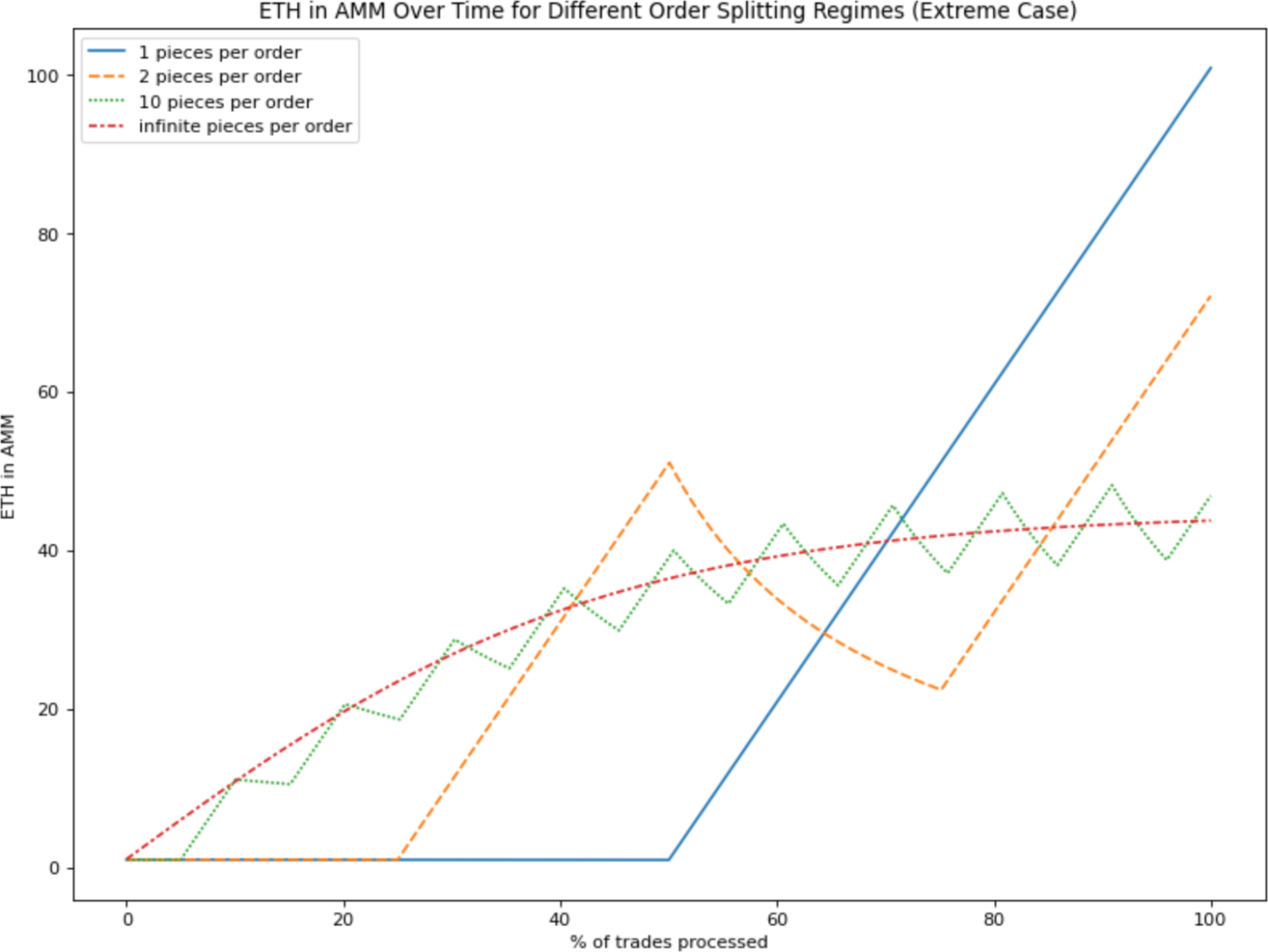## 摘要

TWAMM 通过代 Alice 进行交易，为她解决了这个难题。它将她的订单分解成无限多的极小虚拟订单，以确保随着时间推移完全平稳地执行，并通过与内置 AMM 的特殊数学关系，在这些虚拟订单中分摊 gas 成本。因为它的交易过程是跨区块的，所以也不容易受到三明治攻击的影响。

## 时间加权做市商

### 概述

TWAMM 包含一个内置的 AMM，即这两种资产的标准恒定乘积做市商。任何人都可以在任何时候与这个内置 AMM 进行交易，仿佛它是个普通的 AMM 一样。

TWAMM 将这些长期订单分解为无限多、无限小的虚拟子订单，这些虚拟子订单逐渐以均匀的速度与内置 AMM 交易。单独处理这些虚拟子订单的交易将耗费无限的 gas，但一个闭型数学公式允许我们在需要时才计算其累积结果。

### 基本设计

#### 长期订单

Alice 想在未来 8 小时内买入价值 1 亿美金的 ETH，或者大约 2000 个区块。她在 TWAMM 中下了一个长期订单，在 2000 个区块中购买价值 1 亿 USDC 的 ETH，或每个区块 50,000 USDC。

#### 订单池

Bob 想在接下来的 5000 个区块中卖出 500 个 ETH 换取 USDC，即每个区块 0.1ETH。

Charlie 想在接下来的 2000 个区块中卖出 100 个 ETH 换取 USDC，即每区块 0.05 个 ETH。

### 无限小的虚拟订单

#### 平滑性

TWAMM 的主要目标是在一段时间内平滑地执行长期订单，使其以接近当时的时间加权平均价格执行。## 实现

### 惰性求值

TWAMM 将虚拟子订单视为发生在多个区块之间，这对避免三明治攻击很重要。

### 虚拟交易中的数学

#### 公式

$x_{ammEnd} = \sqrt {\frac {kx_{in}}{y_{in}}}\frac {e^{2\sqrt {\frac {x_{in} y_{in}}{k}}}+c}{e^{2\sqrt {\frac {x_{in} y_{in}}{k}}}-c}$

$c = \frac {\sqrt {x_{ammStart} y_{in}}-\sqrt {y_{ammStart} x_{in}}}{\sqrt {x_{ammStart} y_{in}}+\sqrt {y_{ammStart} x_{in}}}$

$y_{ammEnd}=\frac {x_{ammStart} y_{ammStart}}{x_{ammEnd}}$

X 的卖出池得到所有没有最终进入内置 AMM 的 Y—— 也就是说，

$y_{out} = y_{ammStart} + y_{in} - y_{ammEnd}$

$x_{out} = x_{ammStart} + x_{in} - x_{ammEnd}$